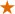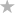#### File Exchange > Graphing >    Gardner-Altman PlotAuthor:
OriginLab Technical Support
8/17/2022
Last Update:
11/3/2022
110
Total Ratings:
2
File Size:
294 KB
Average Rating:File Name:
Gardner-Al...ot.opx
File Version:
1.00
Minimum Versions:
Summary:

Create Gardner–Altman plot with raw or indexed data to compare mean difference.

Description:

PURPOSE
This app can be used to compare mean difference for two groups in raw or indexed data.

INSTALLATION
Download the file Gardner-Altman_Plot.opx, and then drag-and-drop onto the Origin workspace. An icon will appear in the Apps Gallery window.
NOTE: This tool requires OriginPro.

OPERATION

1. Make a worksheet active. Click Gardner-Altman Plot app icon in Apps Gallery window.
2. In the opened dialog, select Data FormatRaw or Indexed. For raw data, choose multiple columns for Raw Data. For indexed data, choose two columns for Data and Factor respectively.
3. In the Settings branch, choose Level as Control, so that other group levels will compare with it. Set a value for Confidence Level (0-1) to estimate mean difference confidence level between two group levels. Specify a value for Number of Bootstrap Samples, and it will be used in the settings for the calculation of bootstrap confidence interval of mean difference. If there are more than two group levels, you can determine whether to Show Gapped Lines between two box plots of two group levels.
4. Click OK button. A Gardner-Altman plot will be created. For data with two group levels, a Double-Y template is used, while Vertical 2 Panel is adopted for more than two group levels.

SAMPLE OPJU FILE
This app provides a sample OPJU file. Right click on the Gardner-Altman Plot icon in the Apps Gallery window, and choose Show Samples Folder from the short-cut menu. A folder will open. Drag-and-drop the project file GardnerAltmanPlotEx.opju from the folder onto Origin. The Notes1 window in the project shows detailed steps.
Note: If you wish to save the OPJU after changing, it is recommended that you save to a different folder location (e.g. User Files Folder).

ALGORITHM
Bias-corrected and accelerated method is used to estimate the bootstrap confidence interval for the mean difference between two group levels.

• Compute the bootstrap mean difference $$\hat{\theta}_b$$.
• Calculate the bias correction $$\hat{Z}_0$$.
• Use jackknife resampling method to calculate the acceleration factor $$\hat{a}$$.
• Correct percents for lower and upper confidence limits with $$\hat{Z}_0$$ and $$\hat{a}$$, for $$100( 1-\alpha )\%$$ confidence interval:
$$\alpha_1 = \Phi( \hat{Z}_0 + \frac{ \hat{Z}_0 + Z_{(\alpha/2)}}{ 1 - \hat{a} ( \hat{Z}_0 + Z_{(\alpha/2)} ) } )$$
$$\alpha_2 = \Phi( \hat{Z}_0 + \frac{ \hat{Z}_0 + Z_{(1-\alpha/2)}}{ 1 - \hat{a} ( \hat{Z}_0 + Z_{(1-\alpha/2)} ) } )$$
where  $$\Phi$$ is the cumulative distribution function of standard normal, and $$Z_{\alpha}$$ is the $$100 \alpha$$-th percentile of standard normal.
• Calculate confidence interval by $$100 \alpha_1$$-th and $$100 \alpha_2$$-th percentiles of $$\hat{\theta}_b$$.

REFERENCE

1. Nathaniel E. Helwig, “Bootstrap Confidence Intervals”, http://users.stat.umn.edu/~helwig/notes/bootci-Notes.pdf
2. DABEST-Python

 01/18/2023 OriginLab Hi, markkramer Thank you for the suggestion. We have reported this in our database and will improve it in the future. OriginLab Technical Service 01/15/2023 markkramer Great app! It would be even better if the user could choose to show the difference in raw units, or in standardised units (e.g., Cohen's d or Hedge's g).